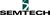##### 5 years ago.

After some time the NAmote gives a "LINK DEAD" indication and appears to stop working.

###### ======== DEBUG STARTED

B: INITFUNC v3 JOINING JOINED Net ID = 0 LED1 = 0 LED1 = 1 TXCOMPLETE LED1 = 0 LED1 = 1 TXCOMPLETE LED1 = 0 LED1 = 1 TXCOMPLETE LED1 = 0 LED1 = 1 TXCOMPLETE LED1 = 0 LED1 = 1 TXCOMPLETE LED1 = 0 LED1 = 1 . . . TXCOMPLETE LED1 = 0 LED1 = 1 TXCOMPLETE LED1 = 0 LED1 = 1 TXCOMPLETE LED1 = 0 LINK_DEAD LED1 = 1

Nothing else after this...

Has this "error" been seen before?

#### Question relating to:LMIC transmit example for NAmote-72 with GPS

It appears to be related to #define CHNL_HYBRID. I am operating with a gateway with a single SX1301 so it was my understanding that I should define CHNL_HYBRID in this configuration. If CHNL_HYBRID is not defined the mote recovers form "LINK DEAD" by re-JOINING.

posted by Greg Davis 24 Nov 2015